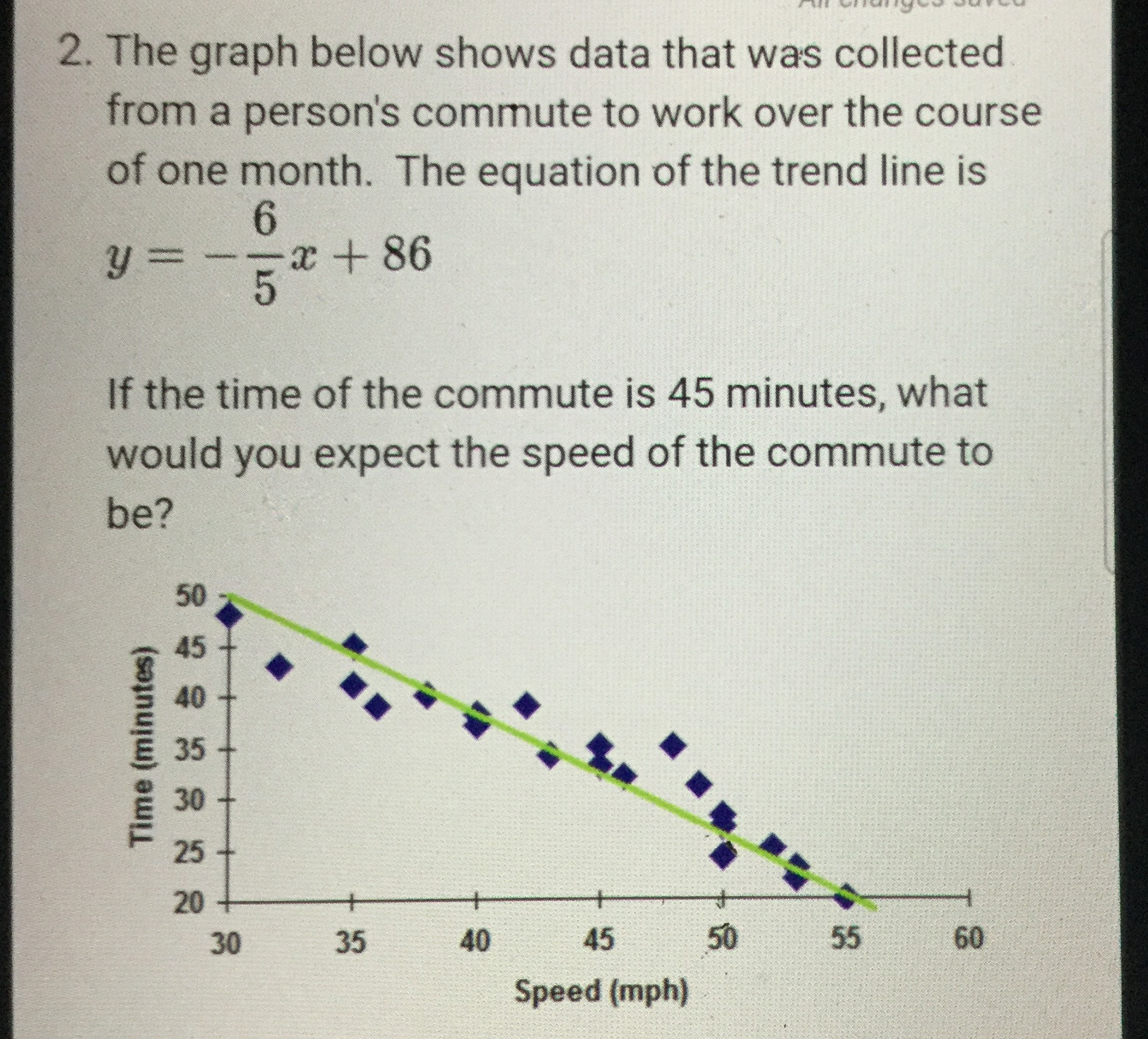### ¿Todavía tienes preguntas de matemáticas?

Pregunte a nuestros tutores expertos
Algebra
Pregunta2. The graph below shows data that was collected from a person's commute to work over the course of one month. The equation of the trend line is

$$y = - \frac { 6 } { 5 } x + 86$$

If the time of the commute is $$45$$ minutes, what would you expect the speed of the commute to be?

$$x = 34.16 \space mph$$